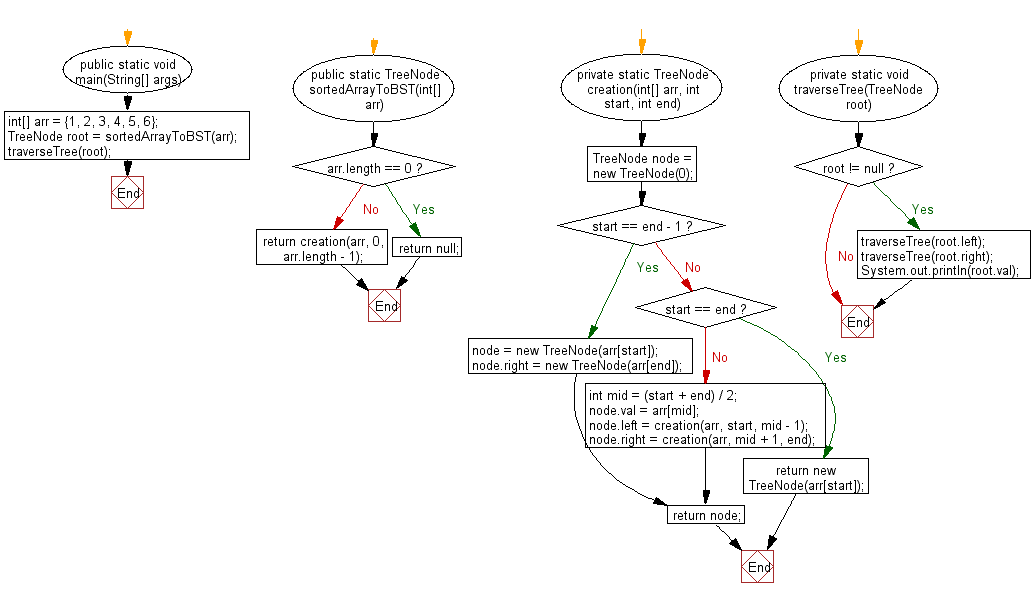﻿ Java exercises: Convert an sorted array to binary search tree - w3resource# Java Exercises: Convert an sorted array to binary search tree

## Java Basic: Exercise-146 with Solution

Write a Java program to convert an sorted array to binary search tree. Maintain minimal height of the tree.

Sample Solution:

Java Code:

``````public class Solution {
public static void main(String[] args) {
int[] arr = {1, 2, 3, 4, 5, 6};
TreeNode root = sortedArrayToBST(arr);
traverseTree(root);
}

public static TreeNode sortedArrayToBST(int[] arr) {
if (arr.length == 0) return null;
return creation(arr, 0, arr.length - 1);
}

private static TreeNode creation(int[] arr, int start, int end) {
TreeNode node = new TreeNode(0);
if (start == end - 1) {
node = new TreeNode(arr[start]);
node.right = new TreeNode(arr[end]);
} else if (start == end) {
return new TreeNode(arr[start]);
} else {
int mid = (start + end) / 2;
node.val = arr[mid];
node.left = creation(arr, start, mid - 1);
node.right = creation(arr, mid + 1, end);
}
return node;
}

private static void traverseTree(TreeNode root) {
if (root != null) {
traverseTree(root.left);
traverseTree(root.right);
System.out.println(root.val);
}
}

}

class TreeNode {
public int val;
public TreeNode left, right;

public TreeNode(int val) {
this.val = val;
this.left = this.right = null;
}
}
```
```

Sample Output:

```2
1
4
6
5
3
```

Flowchart:Java Code Editor:

What is the difficulty level of this exercise?

Test your Programming skills with w3resource's quiz.

﻿

## Java: Tips of the Day

countOccurrences

Counts the occurrences of a value in an array.

Use Arrays.stream().filter().count() to count total number of values that equals the specified value.

```public static long countOccurrences(int[] numbers, int value) {
return Arrays.stream(numbers)
.filter(number -> number == value)
.count();
}
```

Ref: https://bit.ly/3kCAgLb